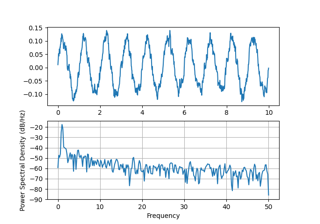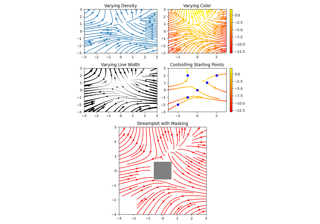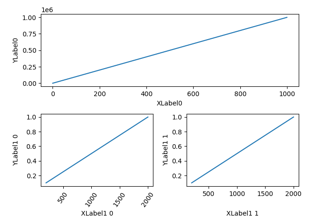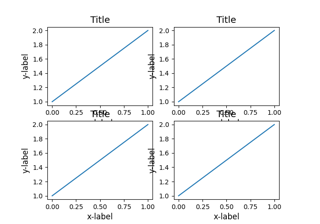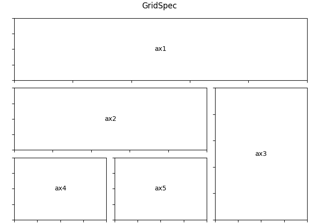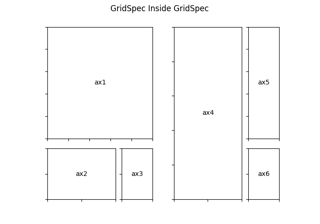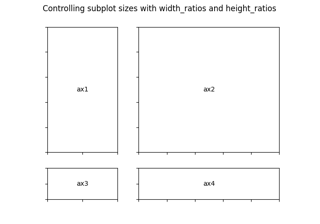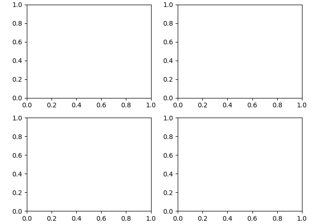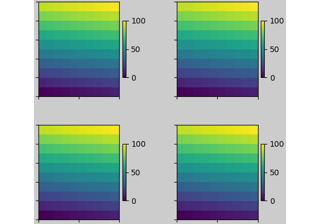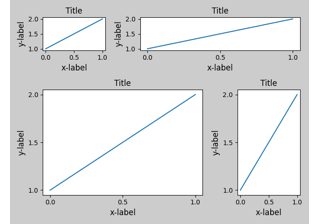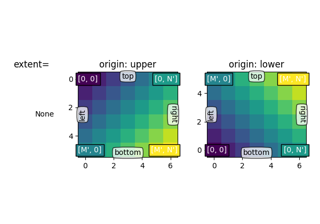# matplotlib.gridspec.GridSpecBase¶

class matplotlib.gridspec.GridSpecBase(nrows, ncols, height_ratios=None, width_ratios=None)[source]

Bases: object

A base class of GridSpec that specifies the geometry of the grid that a subplot will be placed.

Parameters: nrows, ncolsintThe number of rows and columns of the grid. width_ratiosarray-like of length ncols, optionalDefines the relative widths of the columns. Each column gets a relative width of width_ratios[i] / sum(width_ratios). If not given, all columns will have the same width. height_ratiosarray-like of length nrows, optionalDefines the relative heights of the rows. Each column gets a relative height of height_ratios[i] / sum(height_ratios). If not given, all rows will have the same height.
__dict__ = mappingproxy({'__module__': 'matplotlib.gridspec', '__doc__': '\n A base class of GridSpec that specifies the geometry of the grid\n that a subplot will be placed.\n ', '__init__': <function GridSpecBase.__init__>, '__repr__': <function GridSpecBase.__repr__>, 'nrows': <property object>, 'ncols': <property object>, 'get_geometry': <function GridSpecBase.get_geometry>, 'get_subplot_params': <function GridSpecBase.get_subplot_params>, 'new_subplotspec': <function GridSpecBase.new_subplotspec>, 'set_width_ratios': <function GridSpecBase.set_width_ratios>, 'get_width_ratios': <function GridSpecBase.get_width_ratios>, 'set_height_ratios': <function GridSpecBase.set_height_ratios>, 'get_height_ratios': <function GridSpecBase.get_height_ratios>, 'get_grid_positions': <function GridSpecBase.get_grid_positions>, '__getitem__': <function GridSpecBase.__getitem__>, 'subplots': <function GridSpecBase.subplots>, '__dict__': <attribute '__dict__' of 'GridSpecBase' objects>, '__weakref__': <attribute '__weakref__' of 'GridSpecBase' objects>})
__getitem__(self, key)[source]

Create and return a SubplotSpec instance.

__init__(self, nrows, ncols, height_ratios=None, width_ratios=None)[source]
Parameters: nrows, ncolsintThe number of rows and columns of the grid. width_ratiosarray-like of length ncols, optionalDefines the relative widths of the columns. Each column gets a relative width of width_ratios[i] / sum(width_ratios). If not given, all columns will have the same width. height_ratiosarray-like of length nrows, optionalDefines the relative heights of the rows. Each column gets a relative height of height_ratios[i] / sum(height_ratios). If not given, all rows will have the same height.
__module__ = 'matplotlib.gridspec'
__repr__(self)[source]

Return repr(self).

__weakref__

list of weak references to the object (if defined)

get_geometry(self)[source]

Return a tuple containing the number of rows and columns in the grid.

get_grid_positions(self, fig, raw=False)[source]

Return the positions of the grid cells in figure coordinates.

Parameters: figFigureThe figure the grid should be applied to. The subplot parameters (margins and spacing between subplots) are taken from fig. rawbool, default: FalseIf True, the subplot parameters of the figure are not taken into account. The grid spans the range [0, 1] in both directions without margins and there is no space between grid cells. This is used for constrained_layout. bottoms, tops, lefts, rightsarrayThe bottom, top, left, right positions of the grid cells in figure coordinates.
get_height_ratios(self)[source]

Return the height ratios.

This is None if no height ratios have been set explicitly.

get_subplot_params(self, figure=None)[source]
get_width_ratios(self)[source]

Return the width ratios.

This is None if no width ratios have been set explicitly.

property ncols

The number of columns in the grid.

new_subplotspec(self, loc, rowspan=1, colspan=1)[source]

Create and return a SubplotSpec instance.

Parameters: loc(int, int)The position of the subplot in the grid as (row_index, column_index). rowspan, colspanint, default: 1The number of rows and columns the subplot should span in the grid.
property nrows

The number of rows in the grid.

set_height_ratios(self, height_ratios)[source]

Set the relative heights of the rows.

height_ratios must be of length nrows. Each row gets a relative height of height_ratios[i] / sum(height_ratios).

set_width_ratios(self, width_ratios)[source]

Set the relative widths of the columns.

width_ratios must be of length ncols. Each column gets a relative width of width_ratios[i] / sum(width_ratios).

subplots(self, *, sharex=False, sharey=False, squeeze=True, subplot_kw=None)[source]

Add all subplots specified by this GridSpec to its parent figure.

This utility wrapper makes it convenient to create common layouts of subplots in a single call.

Parameters: sharex, shareybool or {'none', 'all', 'row', 'col'}, default: FalseControls sharing of properties among x (sharex) or y (sharey) axes: True or 'all': x- or y-axis will be shared among all subplots. False or 'none': each subplot x- or y-axis will be independent. 'row': each subplot row will share an x- or y-axis. 'col': each subplot column will share an x- or y-axis. When subplots have a shared x-axis along a column, only the x tick labels of the bottom subplot are created. Similarly, when subplots have a shared y-axis along a row, only the y tick labels of the first column subplot are created. To later turn other subplots' ticklabels on, use tick_params. squeezebool, optional, default: True If True, extra dimensions are squeezed out from the returned array of Axes: if only one subplot is constructed (nrows=ncols=1), the resulting single Axes object is returned as a scalar. for Nx1 or 1xM subplots, the returned object is a 1D numpy object array of Axes objects. for NxM, subplots with N>1 and M>1 are returned as a 2D array. If False, no squeezing at all is done: the returned Axes object is always a 2D array containing Axes instances, even if it ends up being 1x1. subplot_kwdict, optionalDict with keywords passed to the add_subplot call used to create each subplot. axAxes object or array of Axes objects.ax can be either a single Axes object or an array of Axes objects if more than one subplot was created. The dimensions of the resulting array can be controlled with the squeeze keyword, see above.

## Examples using matplotlib.gridspec.GridSpecBase¶